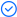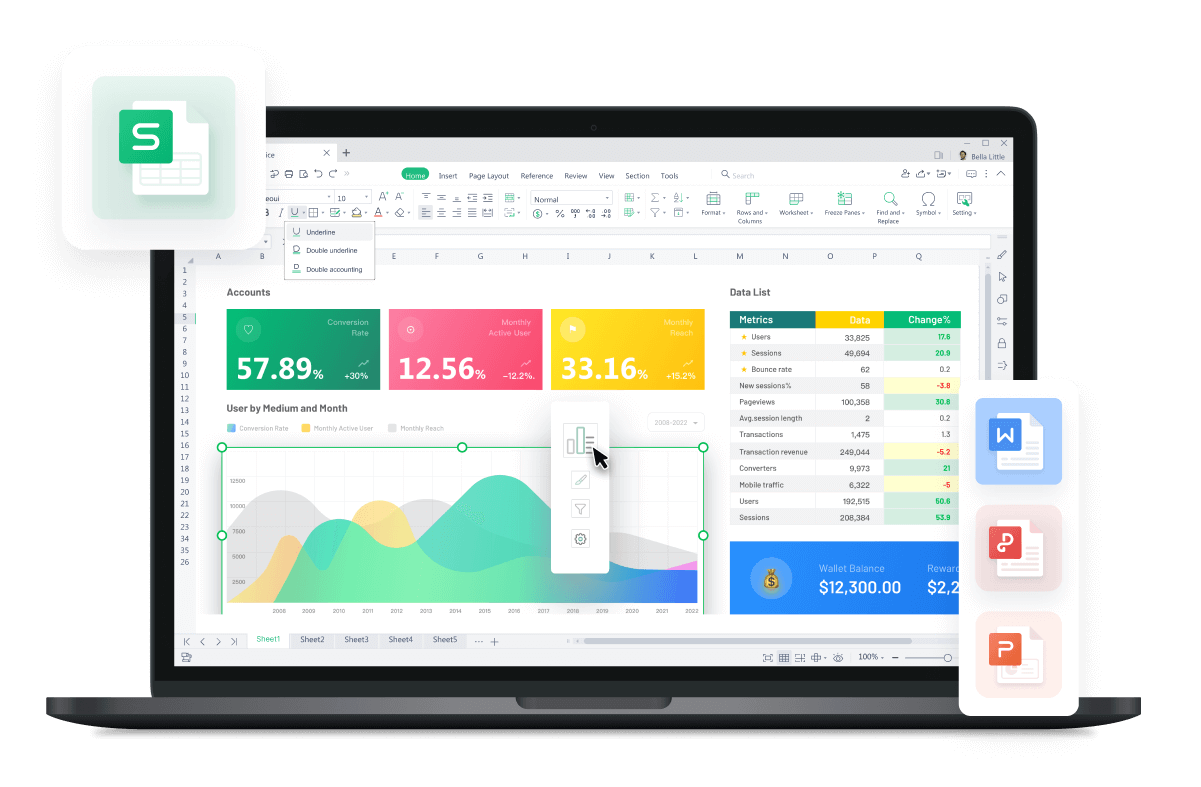WPS Office

Free All-in-One Office Suite with PDF EditorRead, edit, and convert PDFs with the powerful PDF toolkit.Microsoft-like interface, easy to use.

Windows • MacOS • Linux • iOS • Android# LOOKUP Function in Excel

December 17, 2021
6.2K Views

WPS Spreadsheet could be an alternative to Microsoft Office Excel. It includes 100's of built-in formulas, pivot tables, and more.

· Description:

The LOOKUP function can search a value in a column or row and returns a particular value from the same place in another column or row. It has two Syntax.

· Syntax1 :

LOOKUP(value, array)

· Arguments:

Value: The value to search for in an array, which must be in ascending order.

Array:An array of values.(contains both the values to search for and return)

· Example:

Suppose we want to find out the scores of students ranked 1,3,5,7.

2. In this case, we need to enter the LOOKUP Function.

1) Valueis the value to search for in an array, cell G5 is the value that represent the first-ranking student we want to search, so let's enter G5 at Value.

2) Array: An array of values.(contains both the values to search for and return) the area of A3: C12 contains G5 as well as the data we want to return. So let's enter A3:C12 at Array. Also, we need to press F4 to make it an absolute cell reference so that column H6:H8 won't change when copied.

Thus, we input =LOOKUP(G5,\$A\$3:\$C\$12), then press Enter.

The result is 75, which tells us the physics sore of the first-ranking student is 75.

3. Drop-down cell H5 to complete the process.

· Syntax2 :

LOOKUP( value, lookup_vector, [result_vector] )

· Arguments:

Value: The value to search for.

Lookup_vector: A range that contains only one row or one column of texts, numbers, or logical values, placed in ascending order.

Result_vector: [Optional]. Arange that contains only one row or column, the same size as Lookup_vector.

· Example:

Still, suppose we want to find out the scores of students ranked 1,3,5,7. This time we want to use another method to achieve the same end.

2. We need to insert a LOOKUP function:

1) Valueis the value to search for in an array, cell G5 is the value that represent the first-ranking student we want to search, so let's enter G5 at Value.

2) Lookup_vector is the range that contains only one row or one column ofvalues, column A3: A12 is the lookup area that contains cell G5, so let's put C3:C12 here. Also, we need to press F4 to make them absolute cell references so that rows and columns in column H6:H8 won't change when copied.

3) Result_vector is the range that contains only one row or column, the same size as Lookup_vector.In this case, column C3:C12 is the area that tells the physics score that we want to return, so let's put C3:C12 here. Similarly, we need to press F4 to make them absolute cell references so that rows and columns in column H6:H8 won't change when copied.

Thus, we input: =LOOKUP(G5,\$A\$3:\$A\$12,\$C\$3:\$C\$12), then press Enter.

Again, the result is 75, which tells us the physics sore of the first-ranking student is 75 and further verify that our answer is correct.

3. To complete this table,we want to fill the remaining cells in this column, drop-down cell H5.